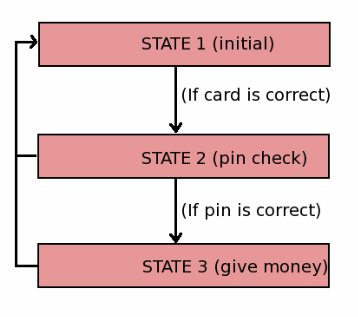black nike shoes roshe cheapnicesports.com

# Sequential Circuits

So far, we have analyzed the importance of gates, and designed circuit using them. The circuit which use only gates (no memory element like flip-flops) are called combinatorial circuits. But there are many applications where the system is not only depends on current input, but also on the sequence of steps performed previously.

For example, take a ATM (automatic teller machine), where first we need to insert our ATM card, then the machine ask's for a secret pin, if pin is correct then only we can withdraw any money from ATM. As you can see the final output (money given out by ATM) depends on the series of sequences.

So the sequential circuit are circuits, where the output to be generated not only depends on the present input but also on the past history of these inputs.

We can divide whole process of withdrawing money in number of stages (or states). In each state the system will check current input and depending on the validity of this input the system may move to next stage. If at any stages the input is not valid, the system will move to initial stage without giving out any money (as shown in the flow chart below).So in order to design any sequential circuit we need few requirements..

1. Some memory element which can hold current state of the system.
2. Some combinatorial logic which will take current state and current input into account to decide what should be the next state.
3. Some combinatorial logic which may be required to decode state into output. This combinatorial logic may use current input as one of the parameter to generate output.

A block diagram of a sequential circuit is shown below